International Journal of Astrophysics and Space Science
Volume 3, Issue 5, October 2015, Pages: 74-77

A Mathematical Aspect of FRW - Cosmological Models Varying t and Λ

A. S. M. Mohiul Islam, Abul Kalam Azad

Department of Mathematics, University of Chittagong, Chittagong, Bangladesh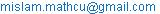(A. S. M. M. Islam)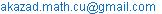(A. K. Azad)

A. S. M. Mohiul Islam, Abul Kalam Azad. A Mathematical Aspect of FRW - Cosmological Models Varying t and Λ.International Journal of Astrophysics and Space Science.Vol.3, No. 5, 2015, pp. 74-77. doi: 10.11648/j.ijass.20150305.12

Abstract: In this paper discussing about the mathematical aspect of variables such as cosmological constant (Λ) and gravitational constant (G) under some suitable assumptions, G(t)=F exp(3Hγt) (where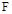is constant and γ is equation of state parameter), and the cosmological constant. Λ∞t-2 The exact solutions for the field equations and discuss some physical properties of the cosmological model. An expanding universe is found by using a relation between Friedmann equation and an equation of state. The cosmological constant has been involved on several occasions to correct some seemingly real difficulty with the cosmological predictions of standard general relativity. The most notable of these where its initial use by Einstein to produce static universe. The physical situation regarding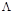is unclear. The other cosmological parameters Hubble constant (H), the density parameter (ρ), Gravitational constant (G) etc, may not constant at all. In this work emphasize to investigate these constants vary with time.

Keywords: Friedmann Model, Cosmological Constant, Inflation, Hubble Parameter

Contents

1. Introduction

"Einstein Universe" is one of Friedmann solutions of Einstein’s field equations for the value of cosmological constant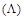. This is only stationary solution of all Friedmann’s solutions, and because it is stationary, it is thought to be non-physical by majority of astronomers. Those astronomers thinks that universe is expanding because there is observed phenomenon of Hubble red shift and it is interpreted by those astronomers as a Doppler’s shift caused by galaxies moving away from our own galaxy. Therefore, it is thought that the real solutions of Einstein’s field equations cannot be stationary. As discussed earlier by many researchers [4, 5] that a constantcannot explain the huge difference between the cosmological constant inferred from observations and energy density resulting from quantum field theories.

The cosmological constantwas introduced such that it was large in the early universe and then decayed with evolution. A number of models with different decay laws for the variation of cosmological term were investigated during last two decades by Abdel-Rahaman 1990  and Berman 1991 , Maharaj and Naido 1993  who obtained cosmological model with time dependent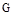andterms, and suggested that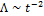.

Many authors [1, 3, 5, 6] have proposed linking of the variation ofwith that ofwithin the rules of general relativity. Mathematically well-posed gravitation theories were developed in which Einstein’s theory of general relativity was generalized to include a varyingby deriving it from Friedmann equation satisfying a conservation equation. There has been considerable interest in solutions of Einstein’s equations of early universe both from cosmological as well as mathematical point of view [1, 5, 6, 7].

A viscous model with variable gravitational and cosmological constant has been considered. The role of viscosity in cosmology has been studied by several authors [1-4]. The bulk viscosity associated with grand unified theory phase transition can lead to the inflationary universe scenario. It is well known that in an early stage of the universe when neutrino decoupling occurred, the matter behaves like viscous fluid .The coefficient of viscosity is known to decrease as the universe expands. Beesham  studied a universe consisting of a cosmological constant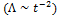and bulk viscosity. More recently Abdel Rahman considered a model in which the gravitational constant G varies with time but energy is conserved [5, 6, 9]. In the present work will investigate the effects of viscosity in a universe vary with time in different cosmological constant like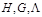etc.

2. The Model

In a Robertson-Walker universe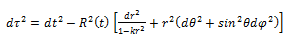(2.1)

where k is the curvature index

Einstein’s field equations with time dependent cosmological and gravitational "constants"(2.2)

and the perfect fluid energy momentum tensor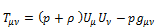(2.3)

yield the two independent equations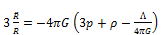(2.4)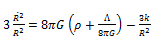(2.5)

Without the cosmological constant solution of equation (2.4) takes the space-space component of Friedmann equation as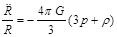(2.6)

and equation(2.5) takes the time-time component of the form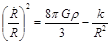(2.7)

For flat universe k=0 and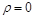, when the matter is not considered as ideal gas in the early universe. Then the equation (2.5) takes the form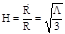where  H=√(Λ/3) is the Hubble Parameter.

Using this statement we get de-sitter universe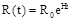(2.8)

This is also the inflationary solution.

From equation (2.4) and (2.5) gives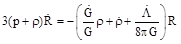(2.9)

The conservation of energy and momentum yields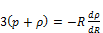(2.10)

The effect of the bulk viscosity in the field equation is to replace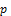by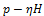, where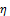is the viscosity co-efficient.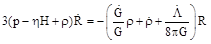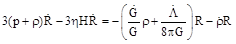It follows immediately that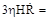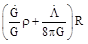(2.11)

and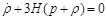(2.12)

Equation (2.11) can also be written as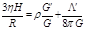(2.13)

Where prime denotes derivative with respect to scale factor R, while dot is the derivative with respect to t. For considering a flat universe, k=0(2.14)

Where(2.14a)and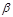= Constant

Also the equation of state is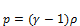(2.15)

Where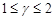In equation (2.12) and (2.15) leads to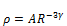(2.16)

where A is the constant of integration.

This is the general solution of density of the universe. If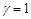, then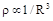is the dust like universe or matter dominant universe  and if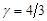, then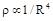is the radiation dominant universe. If, then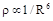for stiff fluid.To distinguish dust and radiation, we will use the symbols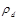and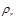to denote their respective densities. Thus in an expanding universe the dust begins to dominate over radiation as the expansion continuous beyond a certain limit. The evidence for ‘dust’ in the form of visible matter in the galaxies, intergalactic medium etc., suggests that at present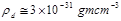(2.17)

From the observational evidence which suggests that the universe may very likely contain dark matter far in execs of the visible matter. It is possible in view of this that the actual matter density in higher. Let us therefore enhance by a dark matter factor,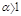and write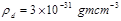(2.18)

The radiation background exists in all observable wavelengths but the most dominant one is in microwaves in the form of a blackbody radiation of temperature ~ 2.7 K, this corresponds to an energy density(2.19)

Thus at present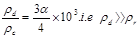As the universe expands this ratio will further increase, not only is the universe matter dominated today, it will continue that way so long as it expands. Clearly for a small enough value of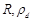was less than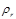.From equation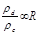and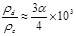we see that this would occur at an epoch of red shift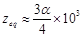(2.20)

at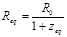The matter and radiation densities were comparable. For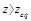and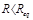, the universe was radiation dominated.

Now from equation (2.14) we have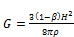(2.21)

Again from equation (2.16) we get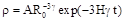Putting the value in equation (2.21) we get finally,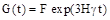where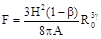=constant

This is another solution of the Gravitational constant  which is not a constant at all but a function of t.

From equation (2.5) and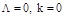and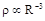and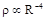, for matter and radiation dominant universe respectively,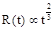and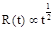From equation (2.5) for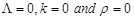then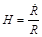for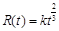and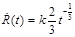And from equation (2.14a) we get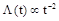Which means the cosmological constant is the function of time and also the inverse square of time. From the above, it can be easily seen that energy density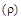and cosmological constantare decreasing while gravitational constant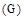is also decreasing during the expansion of the universe.

3. Discussions and Conclusion

This paper to present a variety of classes of solutions to Einstein’s field equations containing different parameters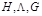, and bulk viscosity. We have listed the forms of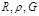and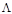as functions of time. It is clear that these quantities depend on the dimensionality of space-time. The cosmological termdecides the behavior of the universe in the model. In this paper we have obtained a negative value ofwhich will corresponds to positive effect mass density. Hence we can get a universe that expands and then reconstruct. The observations on magnitude and red shift that our universe may be an accelerating one or otherwise with induced cosmological density, through the cosmologicalterm [11, 12]. These solutions turned out to be power law, as are these suggested by Bermann . It was shown by Maharaj  that this is also valid for the scalar tensor theory of law k = 0. All power law solutions exhibit the feature of viscous solutions with variable with. We have found that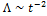for all epochs except the inflationary one.

References

1. Novello, M., and Arauju, R. A. (1980). Phys. Rev. D22, 260.
2. Berman, M. S. (1991). Gen. Rel. Grav.23, 456.
3. Barrow, J. D. (1988).Nucl.Phys.B310, 743.
4. Pimentel, L. O. (1987).Astrophysics. Space Sci. 166, 387.
5. Sistero, M. S. (1991). Gen. Rel. Grav.23, 1265.
6. Abdel Rahaman, A. M. M. (1990). Gen. Rel. Grav. 22,655.
7. Maharaj, S. D.m, and Naido, R. (1993).Astrophysics. Space Sci. 208,261.
8. Klimek, Z. (1976). Nuovo Cimento B35, 249.
9. Kalligas, D., Wesson, P., and Everitt, C. W. (1992). Gen. Rel. Grav. 24,351.
10. Beesham,A.(1993)Phys.Rev. D48, 3539.
11. Islam, J. N.: 2002, An introduction to Mathematical Cosmology, Cambridge Univ. press, Cambridge.
12. Islam, A .S. M. M.: 2015, "Studies on Friedmann model and Cosmological constant", M.Phil thesis, Jamal Nazrul Islam Research center for Mathematical sciences, University of Chittagong.

 Contents 1. 2. 3.
Article ToolsAbstractPDF(176K)